# How to Write Equations & Formulas

Instructor: Michael Quist

Michael has taught college-level mathematics and sociology; high school math, history, science, and speech/drama; and has a doctorate in education.

Writing equations and formulas correctly is generally the first step toward solving or using them, especially in word problems or real life situations. In this lesson, we will explore the steps for writing equations and formulas.

## What are Equations and Formulas?

'We're never going to get there!'

'Yes we are, Tommy. Why don't you do the math? We're traveling at 70 miles per hour, and we're 155 miles away. How long will it take us to get there?'

'I don't know!'

'Well, what's the formula for time traveled, in terms of distance and rate of speed?'

'Oh, you mean the d = rt thing?'

'Right! Now, if you fill in the distance and rate of speed, you can write an equation to solve for how long it will take us to get there.'

'Forget it--wake me up when we get there!'

Solving life's pressing problems is often a matter of finding the right ingredients, setting up the math, and solving.

An equation is math's way of saying that two things are equal to each other--that is, they have the same value, are worth the same amount.

A formula is a special equation that expresses an important relationship between variables and numbers.

## Pieces of an Equation

You can always tell an equation by its equal sign ('='). Equations can have constants, values that are known, as well as variables, these are unknown values typically expressed with letters.

Equation examples: 5 + 6 = 11; x + 4 = 15

Take a look at the image of a simple equation you might see that assigns value to a variable.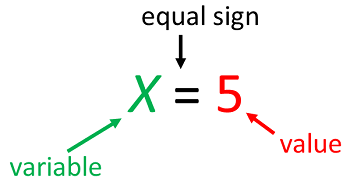Equations don't have to have any numbers at all. For example, you might find that two variables are equal to each other.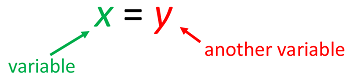Any time you see an equal sign, you know you're looking at an equation. That is the difference between an equation and an expression, which is a mathematical relationship between variables and/or numbers, but without setting the expression equal to something else.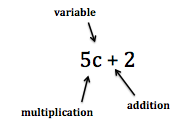We can change an expression into an equation by setting it equal to something.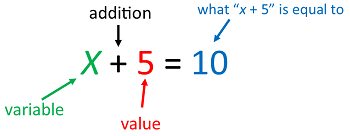## Formulas are Special Equations

A formula has more than one variable and uses these multiple variables to express an important relationship. For example, d = rt is a formula for solving 'distance traveled' problems. Notice that when variables are stuck together with no operation sign between them, that means to multiply them. So, in the d = rt formula, rate of speed (r) and time traveled (t) are multiplied together to get the total distance traveled (d).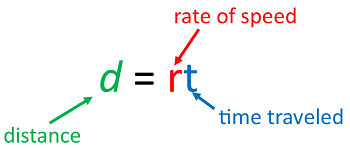## Components of Equations and Formulas

In algebra, equations and formulas are made up of terms , groups of variables and numbers that are connected by multiplication and division. The terms are then tied together by the equal sign and by addition and subtraction operations.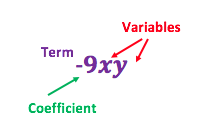Generally, a term may have

• a coefficient (a number that is being multiplied by the rest of the term);
• one or more variables, multiplied together; and
• exponents (number of times that a variable is multiplied by itself).

In 5x², the 5 is multiplied by the rest of the term. An exponent of 2 means that there are actually two x's, being multiplied together. This term means 5 times x times x.

Each side of an equation or formula will have one or more terms, tied together by addition or subtraction. The two sides of the equation or formula will be equal (have the same value).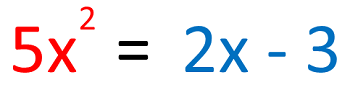## Writing Equations and Formulas from Word Problems

A word problem is a math problem presented in a story format, instead of a written equation. One of the steps in solving a word problem is writing the equation or formula that is appropriate for the situation. For example:

To unlock this lesson you must be a Study.com Member.

### Register to view this lesson

Are you a student or a teacher?

### Unlock Your Education

#### See for yourself why 30 million people use Study.com

##### Become a Study.com member and start learning now.
Back
What teachers are saying about Study.com

### Earning College Credit

Did you know… We have over 160 college courses that prepare you to earn credit by exam that is accepted by over 1,500 colleges and universities. You can test out of the first two years of college and save thousands off your degree. Anyone can earn credit-by-exam regardless of age or education level.